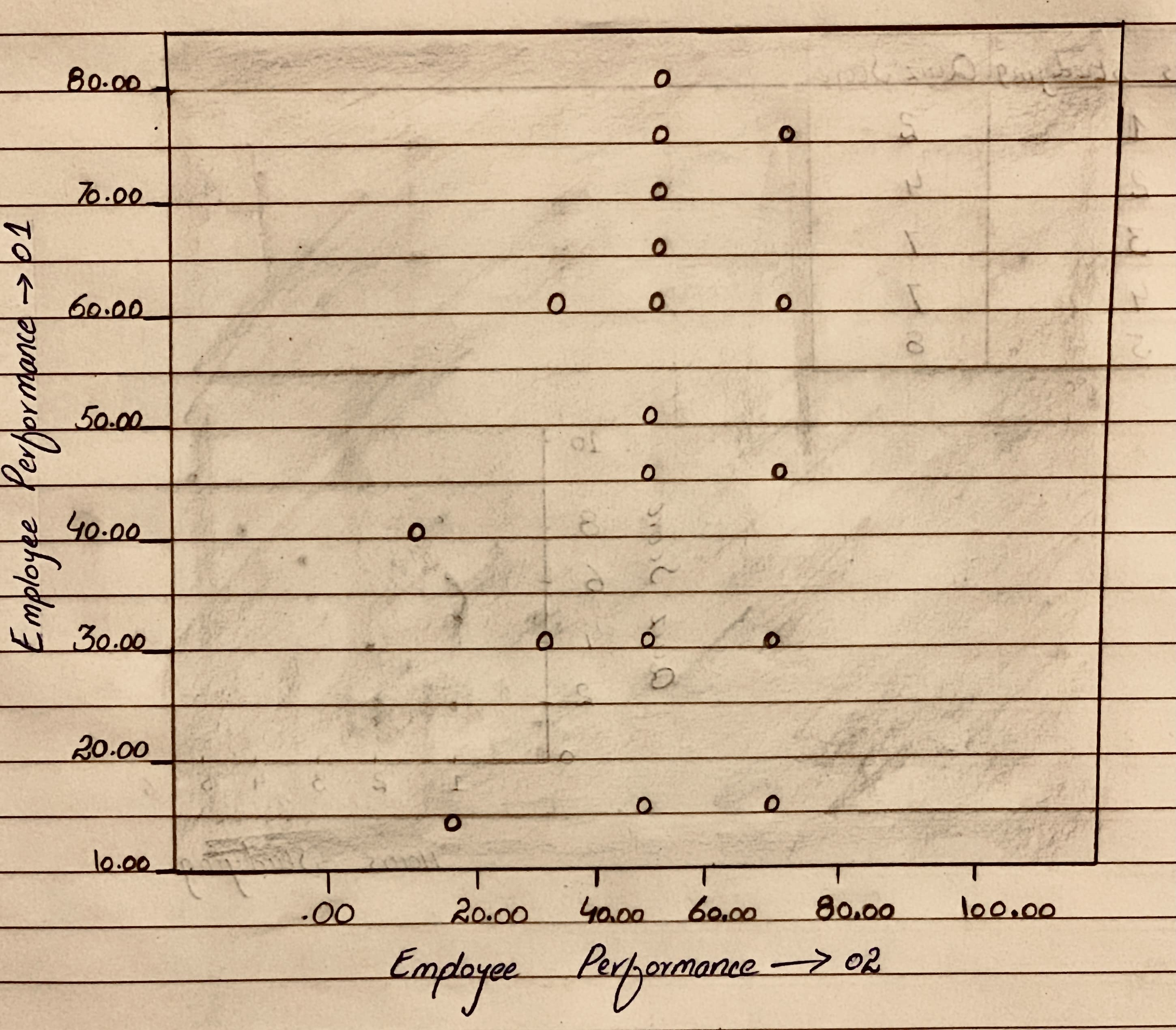# We have to explain the relationship between employee performance 01 and employee performance 02 using the scater plot<img src="13310101191.JPG">pedzenekO 2020-11-02 Answered

We have to explain the relationship between employee performance 01 and employee performance 02 using the scater plotYou can still ask an expert for help

• Questions are typically answered in as fast as 30 minutes

Solve your problem for the price of one coffee

• Math expert for every subject
• Pay only if we can solve itMargot Mill
Step 1
Scatter plot is the graph in which data of the two variables is plotted along the horizontal and vertical axes to determine the relationship between the two variables.
Scatterplots contain a line of best fit, which is a straight line drawn through the center of the data points that best represents the trend of the data.
The closer the data points come when plotted to making a straight line, the higher the correlation between the two variables, or the stronger the relationship.
Step 2
Therefore If there is no apparent relationship between the two variables, then there is no correlation.